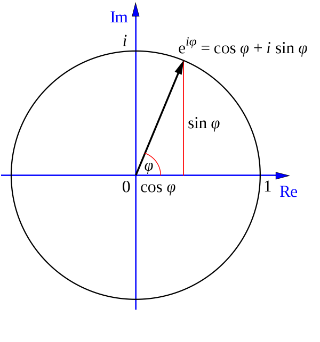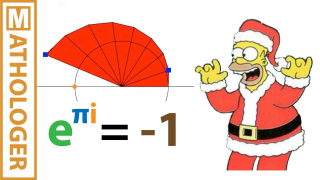# Euler's identity

Topic | v1 | created by jjones |Description

In mathematics, Euler's identity (also known as Euler's equation) is the equality e to the π i = -1, where e is Euler's number, the base of natural logarithms, i is the imaginary unit, which by definition satisfies i² = −1, and π is pi, the ratio of the circumference of a circle to its diameter. Euler's identity is named after the Swiss mathematician Leonhard Euler. It is considered to be an exemplar of mathematical beauty as it shows a profound connection between the most fundamental numbers in mathematics.

##### Resources

For this Christmas video the Mathologer sets out to explain Euler's identity e to the pi i = -1, the...